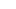Hiak Education Services Pte Ltd

#11-04 Singapore 449408# P4 Watch Out!

October 17, 2017

Area and Perimeter questions will certainly be tested in SA2. There are many types of challenging Area and Perimeter questions that can be tested. We have identified one of them.

<<Concept: Area and Perimeter that involve frames>>

Question: John had a rectangular pool with an area of 48 m². The length of the pool was thrice the breadth of the pool. He decided to build a pebble pathway with a width of 1 m around the pool. What would be the area of the pebble path?

SOLUTION:

Step 1: 48 cm² ÷ 3 = 16 cm² (Explanation: since the length of the pool is 3 times the breath, we can conclude that the area of pool is made up of 3 equal squares of area 16 cm²)

Step 2: 16 cm² = 4 cm x 4 cm (Explanation: If the area of a square is 16 cm², it's side is 4 cm)

Step 3: 4 x 3 cm = 12 cm (Explanation: length of the pool)

Step 4: 12 cm + 1 cm + 1 cm = 14 cm (Explanation: length of the outer area)

Step 5: 4 cm + 1 cm + 1 cm = 6 cm (Explanation: breadth of the outer area)

Step 6: 14 cm x 6 cm = 84 cm² (Explanation:Total outer area)

Step 7: 84 cm² − 48 cm² = 36 cm² (Explanation:Area of pebble path is the difference between the total outer area and the area of the pool)

###### Featured Posts

Factors and Multiples

March 17, 20161/1